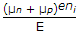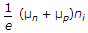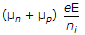# Electronics and Communication Engineering - Electronic Devices and Circuits

11.

Before doping the semiconductor material is

 A. dehydrated B. heated C. hardened D. purified

Answer: Option D

Explanation:

No answer description available for this question. Let us discuss.

12.

The atomic number of silicon is 14. It can be therefore concluded that

 A. a silicon atom contains 14 protons B. a silicon atom contains 14 neutrons C. a silicon atom contains 14 electrons D. all of the above

Answer: Option D

Explanation:

No answer description available for this question. Let us discuss.

13.

X-rays cannot penetrate through a thick sheet of

 A. wood B. paper C. lead D. aluminium

Answer: Option C

Explanation:

No answer description available for this question. Let us discuss.

14.

Ohmic range of metal film resistors is

 A. 1 to 100 ohms B. 10 to 1 K ohms C. 100 to 1 M ohms D. 100 to 100 M ohms

Answer: Option C

Explanation:

No answer description available for this question. Let us discuss.

15.

The current density J, free electron mobility μn, hole mobility μp , magnitude of free electron and hole concentration ni electric field E and charge on electron e, in intrinsic semiconductor are related as

 A. J = (μn + μp)eniE B. J =C. J =D. J =Answer: Option A

Explanation:

No answer description available for this question. Let us discuss.

#### Current Affairs 2021

Interview Questions and Answers